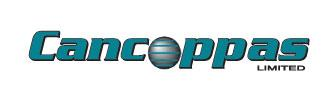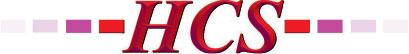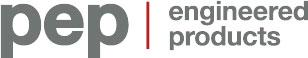AVENSYS.COMCANCOPPAS.COMCGIS.CAEVERESTAUTOMATION.COMFRANKLINEMPIRE.COMHCS1.COMPEP-PETRO.COMSWAGELOK.COMTHERMO-KINETICS.COMTHERMON.COMVANKO.NETVEGA.COMWAJAX.COMWESTECH-IND.COMWIKA.CA

## AutoQuiz: How is the Reynolds Number Used in Flow Measurement?

The post AutoQuiz: How is the Reynolds Number Used in Flow Measurement? first appeared on the ISA Interchange blog site.

AutoQuiz is edited by Joel Don, ISA’s community manager.

Today’s automation industry quiz question comes from the ISA Certified Control Systems Technician (CCST) program. Certified Control System Technicians calibrate, document, troubleshoot, and repair/replace instrumentation for systems that measure and control level, temperature, pressure, flow, and other process variables. Click this link for more information about the CCST program.### This quantity is useful because it describes the ratio between which two quantities?

a) ratio of electrical charge to fluid capacitance for the fluid
b) ratio of specific gravity to heat capacity of the fluid
c) ratio of inertial forces to viscous forces on the fluid
d) ratio of frictional forces to kinetic forces on the fluid
e) none of the above

Answer A cannot be correct: Reynolds number does not indicate a relationship of electrical properties of fluids.

Answer B cannot be correct: Reynolds number does not give a relation to the thermal or mass capacity properties of fluids.

Answer D is not correct. While frictional forces could be equated to viscous forces and kinetic forces could be equated to inertial forces, the ratio indicated in Answer D would be the inverse of the true relationship.

The correct answer is C, the ratio of inertial forces to viscous forces on the fluid.  The inertial forces in the numerator are given by the product of mass (density), fluid velocity, and pipe diameter.  The viscous forces on the fluid are represented by the viscosity in the denominator.

Reference: Hughes, T.A., Measurement and Control Basics, Fifth Edition.Image Source: Wikipedia

Source: ISA News